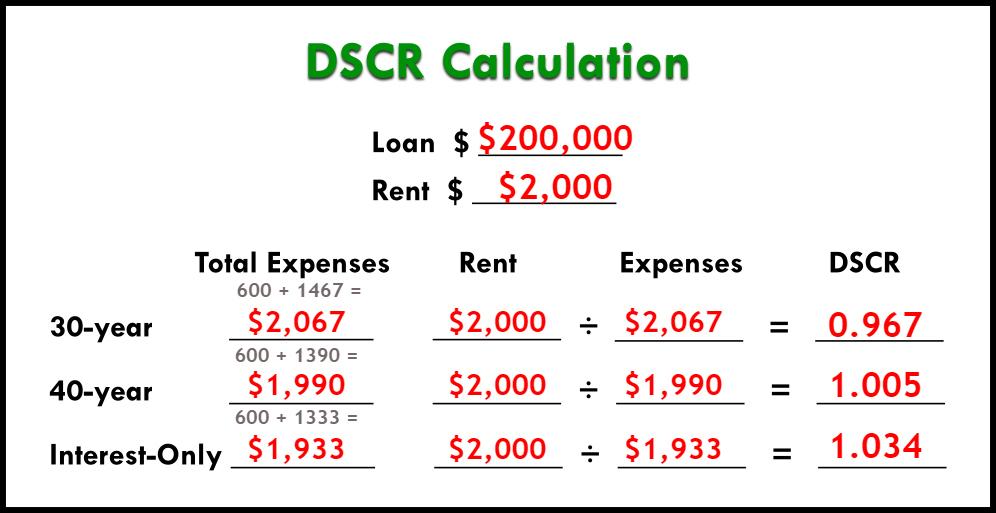# How to Calculate Different DSCR Loan Options

Categories:

There are many DSCR loan options – but how do you calculate the ratio for each one?

Some of your DSCR loan options include 30-year fixed mortgages, 40-year fixed, or interest-only. But how do you know which one’s best?

You’ll have to crunch the numbers. Here’s one example of calculating different DSCR loan options on a \$200,000 loan with \$2,000 rent.

## What Is a DSCR?

DSCR means debt service coverage ratio. It’s a loan for rental properties that hinges on cash flow.

A DSCR loan will be a useful product in your real estate investing career. It requires no income verification and no work or investment history. These loans only require that the property’s income is the same (or higher than) the expenses.

Cash flow is always important to you as an investor, and for DSCR loans, it matters just as much to your lender. The better your cash flow, the better LTV and rates you can get.

It all depends on a little number – the ratio itself. Here’s how to calculate the DSCR with different loan options.

## How to Calculate the DSCR

Loan LTVs and rates on a DSCR are determined by the debt service coverage ratio itself. Now that we have all our raw information, we can plug it into our DSCR calculation to get the ratio.

Here’s how you get the numbers you need:

Add up your expenses (taxes, insurance, and HOA fees) with each loan’s payment amount. Then divide rent by all those expenses.

Costs + Mortgage = Total Expenses

Rent ÷ Total Expenses = DSCR Ratio

Here’s an example of what it would look like with an example using a \$200,000 loan and an 8% interest rate:We want the DSCR to at least equal 1.

Over 1 is ideal. This is a higher cash flow, and you’ll get a better loan.

Less than 1 means negative cash flow, and means you might have to look at a negative DSCR or a no-ratio loan instead.

<1 = Negative Cash Flow

At 1 = Rent = Expenses

>1 = Positive cash flow

Watch the video here.

by